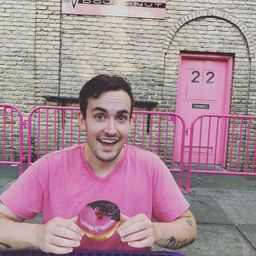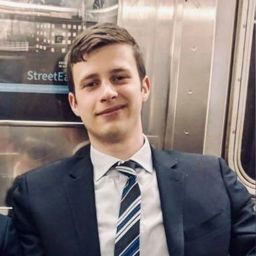Our Discord hit 10K members! 🎉 Meet students and ask top educators your questions.Join Here!

## Educators

HG
MBAS+ 1 more educators

### Problem 1

Ann and Bev have each applied for several jobs at a local university. Let $A$ be the event that Ann is hired and let $B$ be the event that Bev is hired. Express in terms of $A$ and $B$ the events
(a) Ann is hired but not Bev.
(b) At least one of them is hired.
(c) Exactly one of them is hired.

HG
Harsh G.

### Problem 2

Two voters, Al and Bill, are each choosing between one of three candidates-1, $2,$ and $3-$ who are running for city council. An experimental outcome specifies both Al's choice and Bill's choice, e.g., the pair $(3,2)$ .
(a) List all elements of $\mathcal{S}$ .
(b) List all outcomes in the event $A$ that Al and Bill make the same choice.
(c) List all outcomes in the event $B$ that neither of them votes for candidate 2 .

MB
Matt B.

### Problem 3

Four universities $-1,2,3,$ and 4 are participating in a holiday basketball tournament. In the first round, 1 will play 2 and 3 will play $4 .$ Then the two winners will play for the championship, and the two losers will also play. One possible outcome can be denoted by $1324 : 1$ beats 2 and 3 beats 4 in first-round games, and then 1 beats 3 and 2 beats 4
(a) List all outcomes in $\mathcal{S}$ .
(b) Let $A$ denote the event that 1 wins the tournament. List outcomes in $A$ .
(c) Let $B$ denote the event that 2 gets into the championship game. List outcomes in $B$ .
(d) What are the outcomes in $A \cup B$ and in $A \cap B ?$ What are the outcomes in $A^{\prime} ?$Bryan L.

### Problem 4

Suppose that vehicles taking a particular freeway exit can turn right $(R),$ turn left $(L),$ or go straight $(S) .$ Consider observing the direction for each of three successive vehicles.
(a) List all outcomes in the event $A$ that all three vehicles go in the same direction.
(b) List all outcomes in the event $B$ that all three vehicles take different directions.
(c) List all outcomes in the event $C$ that exactly two of the three vehicles turn right.
(d) List all outcomes in the event $D$ that exactly two vehicles go in the same direction.
(e) List the outcomes in $D^{\prime}, C \cup D,$ and $C \cap D .$

MB
Matt B.

### Problem 5

Three components are connected to form a system as shown in the accompanying diagram. Because the components in the $2-3$ subsystem are connected in parallel, that subsystem will function if at least one of the two individual components functions. For the entire system to function, component 1 must function and so must the $2-3$ subsystem.
The experiment consists of determining the condition of each component: $S$ (success) for a
functioning component and $F($ failure) for a nonfunctioning component.
(a) What outcomes are contained in the event $A$ that exactly two out of the three components function?
(b) What outcomes are contained in the event $B$ that at least two of the components function?
(c) What outcomes are contained in the event $C$ that the system functions?
(d) List outcomes in $C^{\prime}, A \cup C, A \cap C, B \cup C,$ and $B \cap C$ .### Problem 6

Each of a sample of four home mortgages is classified as fixed rate $(F)$ or variable rate $(V)$ .
(a) What are the 16 outcomes in $\mathscr{S}$ ?
(b) Which outcomes are in the event that exactly three of the selected mortgages are fixed rate?
(c) Which outcomes are in the event that all four mortgages are of the same type?
(d) Which outcomes are in the event that at most one of the four is a variable-rate mortgage?
(e) What is the union of the events in parts (c) and what is the intersection of these two
events?
(f) What are the union and intersection of the two events in parts (b) and (c)?

MB
Matt B.

### Problem 7

A family consisting of three persons $-A, B,$ and $C-$ belongs to a medical clinic that always has a doctor at each of stations $1,2,$ and $3 .$ During a certain week, each mer of the family visits the clinic once and is assigned at random to a station. The experiment consists of recording the station number for each member. One outcome is $(1,2,1)$ for $A$ to station $1, B$ to station $2,$ and $C$ to station $1 .$
(a) List the 27 outcomes in the sample space.
(b) List all outcomes in the event that all three members go to the same station.
(c) List all outcomes in the event that all members go to different stations.
(d) List all outcomes in the event that no one goes to station 2 .

HG
Harsh G.

### Problem 8

A college library has five copies of a certain text on reserve. Two copies $(1$ and 2$)$ are first printings, and the other three $(3,4,$ and 5$)$ are second printings. A student examines these books in random order, stopping only when a second printing has been selected. One possible outcome is $5,$ and another is $213 .$
(a) List the outcomes in $\mathcal{S}$ .
(b) Let $A$ denote the event that exactly one book must be examined. What outcomes are in $A ?$
(c) Let $B$ be the event that book 5 is the one selected. What outcomes are in $B ?$
(d) Let $C$ be the event that book 1 is not examined. What outcomes are in $C ?$

MB
Matt B.

### Problem 9

An academic department has just completed voting by secret ballot for a department head. The ballot box contains four slips with votes for candidate $A$ and three slips with votes for candidate $B .$ Suppose these slips are removed from the box one by one.
(a) List all possible outcomes.
(b) Suppose a running tally is kept as slips are removed. For what outcomes does $A$ remain ahead of $B$ throughout the tally?

Check back soon!

### Problem 10

A construction firm is currently working on three different buildings. Let $A_{i}$ denote the event that the $i$ th building is completed by the contract date. Use the operations of union, intersection, and complementation to describe each of the following events in terms of $A_{1}, A_{2},$ and $A_{3},$ draw a Venn diagram, and shade the region corresponding to each one.
(a) At least one building is completed by the contract date.
(b) All buildings are completed by the contract date.
(c) Only the first building is completed by the contract date.
(d) Exactly one building is completed by the contract date.
(e) Either the first building or both of the other two buildings are completed by the contract date.

MB
Matt B.

### Problem 11

Use Venn diagrams to verify De Morgan's laws:
(a) $(A \cup B)^{\prime}=A^{\prime} \cap B^{\prime}$
(b) $\quad(A \cap B)^{\prime}=A^{\prime} \cup B^{\prime}$Zechariah R.
(a) In Example $1.10,$ identify three events that are mutually exclusive.
(b) Suppose there is no outcome common to all three of the events $A, B$ , and $C .$ Are these three events necessarily mutually exclusive? If your answer is yes, explain why; if your answer is no, give a counterexample using the experiment of Example $1.10 .$